Do you like the article?
Share it with others -
Use new possibilities of MetaTrader 5

# Timeseries in DoEasy library (part 46): Multi-period multi-symbol indicator buffers

21 October 2020, 13:57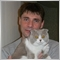0
6 752

### Concept

At the current stage, the library already contains the functionality for creating and tracking multi-period indicator buffers. Now we need to add the functionality for working in the multi-symbol mode so that we are able to handle further tasks aimed at the development of library tools to be used in custom programs.

My previous work with the buffer object classes already provides such a functionality while requiring some refinement. Therefore, today I will make the last preparations and proceed to the simplified development of multi-symbol multi-period standard indicators.
To achieve this, I need to improve the calculated buffer object class so that it is able to accept the array data to its array by the standard indicator handle. The library is capable of doing this already but the small additions I am about to implement greatly facilitate the task and allow for data display on the current chart from standard indicators placed at any symbol/period.

In the following articles, I am going to apply the current concept to the classes for working with standard indicator data from any symbol/period and simplify the task of creating multi-symbol multi-period standard indicators.

### Improving buffer object classes for working with any symbols

The class of the basic abstract buffer object contains the array index values for subsequent indicator buffers that can be created after the current one. In this current object, we have to resort to simple calculations of the indices of subsequent indicator buffers depending on the type of the current buffer object and its drawing style, while the calculation also takes into account the absence of a color buffer for the indicator buffer with the "fill with color between two levels" drawing style.

To simplify the task of calculating the indices of subsequent buffers and not to interfere with the computational clarity when taking into account various factors, we can introduce yet another class member variable containing the number of all arrays used to construct the buffer object. This strictly set value is specified when creating each buffer object (descendant of the abstract buffer class) by passing the necessary value to the protected basic object class constructor in the parameters of the descendant class constructor.

This looks tricky, but in fact everything is very simple: when creating a new indicator buffer object, we already pass some values in the buffer object class constructor to its parent class constructor. Further on, we will pass one more value – the number of arrays necessary for constructing each buffer object.

Open the file of the abstract indicator buffer basic object class \MQL5\Include\DoEasy\Objects\Indicators\Buffer.mqh and make the necessary changes to it.

Declare the new variable in the private section of the class:

```//+------------------------------------------------------------------+
//| Abstract indicator buffer class                                  |
//+------------------------------------------------------------------+
class CBuffer : public CBaseObj
{
private:
long              m_long_prop[BUFFER_PROP_INTEGER_TOTAL];                     // Integer properties
double            m_double_prop[BUFFER_PROP_DOUBLE_TOTAL];                    // Real properties
string            m_string_prop[BUFFER_PROP_STRING_TOTAL];                    // String properties
bool              m_act_state_trigger;                                        // Auxiliary buffer status switch flag
uchar             m_total_arrays;                                             // Total number of buffer arrays

```

When declaring the protected parametric class constructor, add yet another variable in its inputs. This variable is to be used when passing the number of all buffer object arrays to the class while creating this buffer object:

```//--- Default constructor
CBuffer(void){;}
protected:
//--- Protected parametric constructor
CBuffer(ENUM_BUFFER_STATUS status_buffer,
ENUM_BUFFER_TYPE buffer_type,
const uint index_plot,
const uint index_base_array,
const int num_datas,
const uchar total_arrays,
const int width,
const string label);
public:

```

In the constructor implementation code, add this variable to the input list and assign the value passed through it to the previously declared private variable. Then use this value to calculate the basic array index for the next buffer object. When calculating the color array index, check the buffer type. If this is a drawn buffer, calculate the index by adding the number of all data arrays to the basic array index, while in case of the calculated buffer, add zero since the calculated buffer has no color array:

```//+------------------------------------------------------------------+
//| Closed parametric constructor                                    |
//+------------------------------------------------------------------+
CBuffer::CBuffer(ENUM_BUFFER_STATUS buffer_status,
ENUM_BUFFER_TYPE buffer_type,
const uint index_plot,
const uint index_base_array,
const int num_datas,
const uchar total_arrays,
const int width,
const string label)
{
this.m_type=COLLECTION_BUFFERS_ID;
this.m_act_state_trigger=true;
this.m_total_arrays=total_arrays;
//--- Save integer properties
this.m_long_prop[BUFFER_PROP_STATUS]                        = buffer_status;
this.m_long_prop[BUFFER_PROP_TYPE]                          = buffer_type;
ENUM_DRAW_TYPE type=
(
!this.TypeBuffer() || !this.Status() ? DRAW_NONE      :
this.Status()==BUFFER_STATUS_FILLING ? DRAW_FILLING   :
ENUM_DRAW_TYPE(this.Status()+8)
);
this.m_long_prop[BUFFER_PROP_DRAW_TYPE]                     = type;
this.m_long_prop[BUFFER_PROP_TIMEFRAME]                     = PERIOD_CURRENT;
this.m_long_prop[BUFFER_PROP_ACTIVE]                        = true;
this.m_long_prop[BUFFER_PROP_ARROW_CODE]                    = 0x9F;
this.m_long_prop[BUFFER_PROP_ARROW_SHIFT]                   = 0;
this.m_long_prop[BUFFER_PROP_DRAW_BEGIN]                    = 0;
this.m_long_prop[BUFFER_PROP_SHOW_DATA]                     = (buffer_type>BUFFER_TYPE_CALCULATE ? true : false);
this.m_long_prop[BUFFER_PROP_SHIFT]                         = 0;
this.m_long_prop[BUFFER_PROP_LINE_STYLE]                    = STYLE_SOLID;
this.m_long_prop[BUFFER_PROP_LINE_WIDTH]                    = width;
this.m_long_prop[BUFFER_PROP_COLOR_INDEXES]                 = (this.Status()>BUFFER_STATUS_NONE ? (this.Status()!=BUFFER_STATUS_FILLING ? 1 : 2) : 0);
this.m_long_prop[BUFFER_PROP_COLOR]                         = clrRed;
this.m_long_prop[BUFFER_PROP_NUM_DATAS]                     = num_datas;
this.m_long_prop[BUFFER_PROP_INDEX_PLOT]                    = index_plot;
this.m_long_prop[BUFFER_PROP_INDEX_BASE]                    = index_base_array;
this.m_long_prop[BUFFER_PROP_INDEX_COLOR]                   = this.GetProperty(BUFFER_PROP_INDEX_BASE)+
(this.TypeBuffer()!=BUFFER_TYPE_CALCULATE ? this.GetProperty(BUFFER_PROP_NUM_DATAS) : 0);
this.m_long_prop[BUFFER_PROP_INDEX_NEXT_BASE]               = index_base_array+this.m_total_arrays;
this.m_long_prop[BUFFER_PROP_INDEX_NEXT_PLOT]               = (this.TypeBuffer()>BUFFER_TYPE_CALCULATE ? index_plot+1 : index_plot);

//--- Save real properties
this.m_double_prop[this.IndexProp(BUFFER_PROP_EMPTY_VALUE)] = (this.TypeBuffer()>BUFFER_TYPE_CALCULATE ? EMPTY_VALUE : 0);
//--- Save string properties
this.m_string_prop[this.IndexProp(BUFFER_PROP_SYMBOL)]      = ::Symbol();
this.m_string_prop[this.IndexProp(BUFFER_PROP_LABEL)]       = (this.TypeBuffer()>BUFFER_TYPE_CALCULATE ? label : NULL);

//--- If failed to change the size of the indicator buffer array, display the appropriate message indicating the string
if(::ArrayResize(this.DataBuffer,(int)this.GetProperty(BUFFER_PROP_NUM_DATAS))==WRONG_VALUE)
::Print(DFUN_ERR_LINE,CMessage::Text(MSG_LIB_SYS_FAILED_DRAWING_ARRAY_RESIZE),". ",CMessage::Text(MSG_LIB_SYS_ERROR),": ",(string)::GetLastError());

//--- If failed to change the size of the color array (only for a non-calculated buffer), display the appropriate message indicating the string
if(this.TypeBuffer()>BUFFER_TYPE_CALCULATE)
if(::ArrayResize(this.ArrayColors,(int)this.ColorsTotal())==WRONG_VALUE)
::Print(DFUN_ERR_LINE,CMessage::Text(MSG_LIB_SYS_FAILED_COLORS_ARRAY_RESIZE),". ",CMessage::Text(MSG_LIB_SYS_ERROR),": ",(string)::GetLastError());

//--- For DRAW_FILLING, fill in the color array with two default colors
if(this.Status()==BUFFER_STATUS_FILLING)
{
this.SetColor(clrBlue,0);
this.SetColor(clrRed,1);
}

//--- Bind indicator buffers with arrays
//--- In a loop by the number of indicator buffers
int total=::ArraySize(DataBuffer);
for(int i=0;i<total;i++)
{
//--- calculate the index of the next array and
//--- bind the indicator buffer by the calculated index with the dynamic array
//--- located by the i loop index in the DataBuffer array
int index=(int)this.GetProperty(BUFFER_PROP_INDEX_BASE)+i;
::SetIndexBuffer(index,this.DataBuffer[i].Array,(this.TypeBuffer()==BUFFER_TYPE_DATA ? INDICATOR_DATA : INDICATOR_CALCULATIONS));
//--- Set indexation flag as in the timeseries to all buffer arrays
::ArraySetAsSeries(this.DataBuffer[i].Array,true);
}
//--- Bind the color buffer with the array (only for a non-calculated buffer and not for the filling buffer)
if(this.Status()!=BUFFER_STATUS_FILLING && this.TypeBuffer()!=BUFFER_TYPE_CALCULATE)
{
::SetIndexBuffer((int)this.GetProperty(BUFFER_PROP_INDEX_COLOR),this.ColorBufferArray,INDICATOR_COLOR_INDEX);
::ArraySetAsSeries(this.ColorBufferArray,true);
}
//--- If this is a calculated buffer, all is done
if(this.TypeBuffer()==BUFFER_TYPE_CALCULATE)
return;
//--- Set integer parameters of the graphical series
::PlotIndexSetInteger((int)this.GetProperty(BUFFER_PROP_INDEX_PLOT),PLOT_DRAW_TYPE,(ENUM_PLOT_PROPERTY_INTEGER)this.GetProperty(BUFFER_PROP_DRAW_TYPE));
::PlotIndexSetInteger((int)this.GetProperty(BUFFER_PROP_INDEX_PLOT),PLOT_ARROW,(ENUM_PLOT_PROPERTY_INTEGER)this.GetProperty(BUFFER_PROP_ARROW_CODE));
::PlotIndexSetInteger((int)this.GetProperty(BUFFER_PROP_INDEX_PLOT),PLOT_ARROW_SHIFT,(ENUM_PLOT_PROPERTY_INTEGER)this.GetProperty(BUFFER_PROP_ARROW_SHIFT));
::PlotIndexSetInteger((int)this.GetProperty(BUFFER_PROP_INDEX_PLOT),PLOT_DRAW_BEGIN,(ENUM_PLOT_PROPERTY_INTEGER)this.GetProperty(BUFFER_PROP_DRAW_BEGIN));
::PlotIndexSetInteger((int)this.GetProperty(BUFFER_PROP_INDEX_PLOT),PLOT_SHOW_DATA,(ENUM_PLOT_PROPERTY_INTEGER)this.GetProperty(BUFFER_PROP_SHOW_DATA));
::PlotIndexSetInteger((int)this.GetProperty(BUFFER_PROP_INDEX_PLOT),PLOT_SHIFT,(ENUM_PLOT_PROPERTY_INTEGER)this.GetProperty(BUFFER_PROP_SHIFT));
::PlotIndexSetInteger((int)this.GetProperty(BUFFER_PROP_INDEX_PLOT),PLOT_LINE_STYLE,(ENUM_PLOT_PROPERTY_INTEGER)this.GetProperty(BUFFER_PROP_LINE_STYLE));
::PlotIndexSetInteger((int)this.GetProperty(BUFFER_PROP_INDEX_PLOT),PLOT_LINE_WIDTH,(ENUM_PLOT_PROPERTY_INTEGER)this.GetProperty(BUFFER_PROP_LINE_WIDTH));
this.SetColor((color)this.GetProperty(BUFFER_PROP_COLOR));
//--- Set real parameters of the graphical series
::PlotIndexSetDouble((int)this.GetProperty(BUFFER_PROP_INDEX_PLOT),PLOT_EMPTY_VALUE,this.GetProperty(BUFFER_PROP_EMPTY_VALUE));
//--- Set string parameters of the graphical series
::PlotIndexSetString((int)this.GetProperty(BUFFER_PROP_INDEX_PLOT),PLOT_LABEL,this.GetProperty(BUFFER_PROP_LABEL));
}
//+------------------------------------------------------------------+

```

Now all classes of descendant objects of the abstract buffer basic object are supplemented with passing the required number of used arrays for constructing the buffer in the initialization list of class constructors.

For the array buffer (\MQL5\Include\DoEasy\Objects\Indicators\BufferArrow.mqh):

```//+------------------------------------------------------------------+
//| Buffer with the "Drawing with arrows" drawing style              |
//+------------------------------------------------------------------+
class CBufferArrow : public CBuffer
{
private:

public:
//--- Constructor
CBufferArrow(const uint index_plot,const uint index_base_array) :
CBuffer(BUFFER_STATUS_ARROW,BUFFER_TYPE_DATA,index_plot,index_base_array,1,2,1,"Arrows") {}

```

For the line buffer (\MQL5\Include\DoEasy\Objects\Indicators\BufferLine.mqh):

```//+------------------------------------------------------------------+
//| Buffer of the Line drawing style                                 |
//+------------------------------------------------------------------+
class CBufferLine : public CBuffer
{
private:

public:
//--- Constructor
CBufferLine(const uint index_plot,const uint index_base_array) :
CBuffer(BUFFER_STATUS_LINE,BUFFER_TYPE_DATA,index_plot,index_base_array,1,2,1,"Line") {}

```

For the section buffer (\MQL5\Include\DoEasy\Objects\Indicators\BufferSection.mqh):

```//+------------------------------------------------------------------+
//| Buffer of the Section drawing style                              |
//+------------------------------------------------------------------+
class CBufferSection : public CBuffer
{
private:

public:
//--- Constructor
CBufferSection(const uint index_plot,const uint index_base_array) :
CBuffer(BUFFER_STATUS_SECTION,BUFFER_TYPE_DATA,index_plot,index_base_array,1,2,1,"Section") {}

```

For the histogram buffer from the zero line (\MQL5\Include\DoEasy\Objects\Indicators\BufferHistogram.mqh):

```//+------------------------------------------------------------------+
//| Buffer of the "Histogram from the zero line" drawing style       |
//+------------------------------------------------------------------+
class CBufferHistogram : public CBuffer
{
private:

public:
//--- Constructor
CBufferHistogram(const uint index_plot,const uint index_base_array) :
CBuffer(BUFFER_STATUS_HISTOGRAM,BUFFER_TYPE_DATA,index_plot,index_base_array,1,2,2,"Histogram") {}

```

For the histogram buffer on two indicator buffers (\MQL5\Include\DoEasy\Objects\Indicators\BufferHistogram2.mqh):

```//+--------------------------------------------------------------------+
//|Buffer of the "Histogram on two indicator buffers" drawing style    |
//+--------------------------------------------------------------------+
class CBufferHistogram2 : public CBuffer
{
private:

public:
//--- Constructor
CBufferHistogram2(const uint index_plot,const uint index_base_array) :
CBuffer(BUFFER_STATUS_HISTOGRAM2,BUFFER_TYPE_DATA,index_plot,index_base_array,2,3,8,"Histogram2 0;Histogram2 1") {}

```

For the zigzag buffer (\MQL5\Include\DoEasy\Objects\Indicators\BufferZigZag.mqh):

```//+------------------------------------------------------------------+
//|Buffer of the ZigZag drawing style                                |
//+------------------------------------------------------------------+
class CBufferZigZag : public CBuffer
{
private:

public:
//--- Constructor
CBufferZigZag(const uint index_plot,const uint index_base_array) :
CBuffer(BUFFER_STATUS_ZIGZAG,BUFFER_TYPE_DATA,index_plot,index_base_array,2,3,1,"ZigZag 0;ZigZag 1") {}

```

For the filling buffer (\MQL5\Include\DoEasy\Objects\Indicators\BufferFilling.mqh):

```//+------------------------------------------------------------------+
//|Buffer of the "Color filling between two levels" drawing style    |
//+------------------------------------------------------------------+
class CBufferFilling : public CBuffer
{
private:

public:
//--- Constructor
CBufferFilling(const uint index_plot,const uint index_base_array) :
CBuffer(BUFFER_STATUS_FILLING,BUFFER_TYPE_DATA,index_plot,index_base_array,2,2,1,"Filling 0;Filling 1") {}

```

For the buffer of drawing as bars (\MQL5\Include\DoEasy\Objects\Indicators\BufferBars.mqh):

```//+------------------------------------------------------------------+
//|Buffer of the Bars drawing style                                  |
//+------------------------------------------------------------------+
class CBufferBars : public CBuffer
{
private:

public:
//--- Constructor
CBufferBars(const uint index_plot,const uint index_base_array) :
CBuffer(BUFFER_STATUS_BARS,BUFFER_TYPE_DATA,index_plot,index_base_array,4,5,2,"Bar Open;Bar High;Bar Low;Bar Close") {}

```

For the buffer of drawing as candles (\MQL5\Include\DoEasy\Objects\Indicators\BufferCandles.mqh):

```//+------------------------------------------------------------------+
//|Buffer of the Candles drawing style                               |
//+------------------------------------------------------------------+
class CBufferCandles : public CBuffer
{
private:

public:
//--- Constructor
CBufferCandles(const uint index_plot,const uint index_base_array) :
CBuffer(BUFFER_STATUS_CANDLES,BUFFER_TYPE_DATA,index_plot,index_base_array,4,5,1,"Candle Open;Candle High;Candle Low;Candle Close") {}

```

For the calculated buffer (\MQL5\Include\DoEasy\Objects\Indicators\BufferCalculate.mqh):

```//+------------------------------------------------------------------+
//| Calculated buffer                                                |
//+------------------------------------------------------------------+
class CBufferCalculate : public CBuffer
{
private:

public:
//--- Constructor
CBufferCalculate(const uint index_plot,const uint index_array) :
CBuffer(BUFFER_STATUS_NONE,BUFFER_TYPE_CALCULATE,index_plot,index_array,1,1,0,"Calculate") {}

```

Such changes relieve us of the need to perform checks for the buffer type and drawing style in order to calculate indices of subsequent created buffers, since the same number of arrays is always used for each buffer type — it is passed in the form of strictly specified values when creating a buffer.

In the calculated buffer class, add new methods to write data from the standard indicator handle to the calculated buffer array:

```//+------------------------------------------------------------------+
//| Calculated buffer                                                |
//+------------------------------------------------------------------+
class CBufferCalculate : public CBuffer
{
private:

public:
//--- Constructor
CBufferCalculate(const uint index_plot,const uint index_array) :
CBuffer(BUFFER_STATUS_NONE,BUFFER_TYPE_CALCULATE,index_plot,index_array,1,1,0,"Calculate") {}
//--- Supported integer properties of a buffer
virtual bool      SupportProperty(ENUM_BUFFER_PROP_INTEGER property);
//--- Supported real properties of a buffer
virtual bool      SupportProperty(ENUM_BUFFER_PROP_DOUBLE property);
//--- Supported string properties of a buffer
virtual bool      SupportProperty(ENUM_BUFFER_PROP_STRING property);
//--- Display a short buffer description in the journal
virtual void      PrintShort(void);

//--- Set the value to the data buffer array
void              SetData(const uint series_index,const double value)               { this.SetBufferValue(0,series_index,value);       }
//--- Return the value from the data buffer array
double            GetData(const uint series_index)                            const { return this.GetDataBufferValue(0,series_index);  }

//--- Copy data of the specified indicator to the buffer object array
int               FillAsSeries(const int indicator_handle,const int buffer_num,const int start_pos,const int count);
int               FillAsSeries(const int indicator_handle,const int buffer_num,const datetime start_time,const int count);
int               FillAsSeries(const int indicator_handle,const int buffer_num,const datetime start_time,const datetime stop_time);

};
//+------------------------------------------------------------------+

```

Let's write their implementation outside the class body:

```//+------------------------------------------------------------------+
//| Copy data of the specified indicator to the buffer object array  |
//+------------------------------------------------------------------+
int CBufferCalculate::FillAsSeries(const int indicator_handle,const int buffer_num,const int start_pos,const int count)
{
return ::CopyBuffer(indicator_handle,buffer_num,start_pos,count,this.DataBuffer.Array);
}
//+------------------------------------------------------------------+
int CBufferCalculate::FillAsSeries(const int indicator_handle,const int buffer_num,const datetime start_time,const int count)
{
return ::CopyBuffer(indicator_handle,buffer_num,start_time,count,this.DataBuffer.Array);
}
//+------------------------------------------------------------------+
int CBufferCalculate::FillAsSeries(const int indicator_handle,const int buffer_num,const datetime start_time,const datetime stop_time)
{
return ::CopyBuffer(indicator_handle,buffer_num,start_time,stop_time,this.DataBuffer.Array);
}
//+------------------------------------------------------------------+
```

All three methods use three variants of the CopyBuffer() overloaded function. The array assigned by the appropriate indicator buffer is used as a receiving array. The appropriate indicator buffer is the one the methods for writing the necessary indicator data by its handle to the object array are called from.

Now let's implement the multi-symbol mode of working with buffer objects. First, I need to address some of my assumptions I made when preparing the material for the previous article, in which I implemented the multi-period mode.
In the indicator buffer collection class, I have created the method for receiving the necessary timeseries and bar data for working with a single buffer bar. This method features all the necessary data on chart periods — the current and assigned buffer object, as well as all the necessary data on symbols — the current and assigned buffer object. Below is the method from the previous article:

```//+------------------------------------------------------------------+
//| Get data of the necessary timeseries and bars                    |
//| for working with a single bar of the buffer                      |
//+------------------------------------------------------------------+
int CBuffersCollection::GetBarsData(CBuffer *buffer,const int series_index,int &index_bar_period)
{
//--- Get timeseries of the current chart and the chart of the buffer timeframe
CSeriesDE *series_current=this.m_timeseries.GetSeries(buffer.Symbol(),PERIOD_CURRENT);
CSeriesDE *series_period=this.m_timeseries.GetSeries(buffer.Symbol(),buffer.Timeframe());
if(series_current==NULL || series_period==NULL)
return WRONG_VALUE;
//--- Get the bar object of the current timeseries corresponding to the required timeseries index
CBar *bar_current=series_current.GetBar(series_index);
if(bar_current==NULL)
return WRONG_VALUE;
//--- Get the timeseries bar object of the buffer chart period corresponding to the time the timeseries bar of the current chart falls into
CBar *bar_period=m_timeseries.GetBarSeriesFirstFromSeriesSecond(NULL,PERIOD_CURRENT,bar_current.Time(),NULL,series_period.Timeframe());
if(bar_period==NULL)
return WRONG_VALUE;
//--- Write down the bar index on the current timeframe which falls into the bar start time of the buffer object chart
index_bar_period=bar_period.Index(PERIOD_CURRENT);
//--- Calculate the amount of bars of the current timeframe included into one bar of the buffer object chart period
//--- and return this value (1 if the result is 0)
int num_bars=::PeriodSeconds(bar_period.Timeframe())/::PeriodSeconds(bar_current.Timeframe());
return(num_bars>0 ? num_bars : 1);
}
//+------------------------------------------------------------------+

```

Here I have missed getting data from the necessary symbol in just two strings — for the current chart timeseries, we got data from the chart of the symbol assigned to the buffer object. The case is quite the opposite in the second string where we get the current chart symbol at the point where we need to take the buffer object symbol.

As a result, all corrections boil down to only two fixes in the two code strings.

The full listing of the fixed method:

```//+------------------------------------------------------------------+
//| Get data of the necessary timeseries and bars                    |
//| for working with a single bar of the buffer                      |
//+------------------------------------------------------------------+
int CBuffersCollection::GetBarsData(CBuffer *buffer,const int series_index,int &index_bar_period)
{
//--- Get timeseries of the current chart and the chart of the buffer timeframe
CSeriesDE *series_current=this.m_timeseries.GetSeries(Symbol(),PERIOD_CURRENT);
CSeriesDE *series_period=this.m_timeseries.GetSeries(buffer.Symbol(),buffer.Timeframe());
if(series_current==NULL || series_period==NULL)
return WRONG_VALUE;
//--- Get the bar object of the current timeseries corresponding to the required timeseries index
CBar *bar_current=series_current.GetBar(series_index);
if(bar_current==NULL)
return WRONG_VALUE;
//--- Get the timeseries bar object of the buffer chart period corresponding to the time the timeseries bar of the current chart falls into
CBar *bar_period=m_timeseries.GetBarSeriesFirstFromSeriesSecond(NULL,PERIOD_CURRENT,bar_current.Time(),buffer.Symbol(),series_period.Timeframe());
if(bar_period==NULL)
return WRONG_VALUE;
//--- Write down the bar index on the current timeframe which falls into the bar start time of the buffer object chart
index_bar_period=bar_period.Index(PERIOD_CURRENT);
//--- Calculate the amount of bars of the current timeframe included into one bar of the buffer object chart period
//--- and return this value (1 if the result is 0)
int num_bars=::PeriodSeconds(bar_period.Timeframe())/::PeriodSeconds(bar_current.Timeframe());
return(num_bars>0 ? num_bars : 1);
}
//+------------------------------------------------------------------+

```

All is set. Now our buffer objects are able to work in the multi-symbol mode as well.

We still lack the method that will return the bar index on a symbol/period chart the index of the specified bar of the current chart falls into. The method is necessary for correct display of data from another symbol/period on the current chart during the main indicator loop.

The most suitable place for such a method is the timeseries collection class \MQL5\Include\DoEasy\Collections\TimeSeriesCollection.mqh.
Declare the new method in it:

```//--- Return the bar object of the specified timeseries of the specified symbol of the specified position (1) by index, (2) by time
//--- bar object of the first timeseries corresponding to the bar open time on the second timeseries (3) by index, (4) by time
CBar                   *GetBar(const string symbol,const ENUM_TIMEFRAMES timeframe,const int index,const bool from_series=true);
CBar                   *GetBar(const string symbol,const ENUM_TIMEFRAMES timeframe,const datetime bar_time);
CBar                   *GetBarSeriesFirstFromSeriesSecond(const string symbol_first,const ENUM_TIMEFRAMES timeframe_first,const int index,
const string symbol_second=NULL,const ENUM_TIMEFRAMES timeframe_second=PERIOD_CURRENT);
CBar                   *GetBarSeriesFirstFromSeriesSecond(const string symbol_first,const ENUM_TIMEFRAMES timeframe_first,const datetime first_bar_time,
const string symbol_second=NULL,const ENUM_TIMEFRAMES timeframe_second=PERIOD_CURRENT);

//--- Return the bar index on the specified timeframe chart by the current chart's bar index                                |
int                     IndexBarPeriodByBarCurrent(const int series_index,const string symbol,const ENUM_TIMEFRAMES timeframe);

//--- Return the flag of opening a new bar of the specified timeseries of the specified symbol
bool                    IsNewBar(const string symbol,const ENUM_TIMEFRAMES timeframe,const datetime time=0);

```

Let's write its implementation outside the class body:

```//+------------------------------------------------------------------+
//| Return the bar index on the specified timeframe chart            |
//| by the current chart's bar index                                 |
//+------------------------------------------------------------------+
int CTimeSeriesCollection::IndexBarPeriodByBarCurrent(const int series_index,const string symbol,const ENUM_TIMEFRAMES timeframe)
{
CSeriesDE *series=this.GetSeries(::Symbol(),(ENUM_TIMEFRAMES)::Period());
if(series==NULL)
return WRONG_VALUE;
CBar *bar=series.GetBar(series_index);
if(bar==NULL)
return WRONG_VALUE;
return ::iBarShift(symbol,timeframe,bar.Time());
}
//+------------------------------------------------------------------+
```

The method receives the current chart bar index, as well as a symbol and period of the chart, for which the bar index corresponding to the time of the current chart index passed to the method should be returned.

Further on, we get the pointer to the current chart timeseries, get the pointer to the bar object by the current timeseries index and use the bar time to return the index of the corresponding bar on the required timeseries.

Since the calculated buffer is to store the indicator buffer data in full accordance with the timeseries the indicator is based on, this method is used to get the index in the calculated buffer array corresponding to the specified bar index on the current chart (the indicator loop index can be used as a practical example here). If we can establish such a matching between two various timeseries, then we can correctly display these data on the necessary chart.

To access the method from custom programs, we need to provide access to it from the CEngine library main object class (\MQL5\Include\DoEasy\Engine.mqh):

```//--- Clear data by the timeseries index for the (1) arrow, (2) line, (3) section, (4) zero line histogram,
//--- (5) histogram on two buffers, (6) zigzag, (7) filling, (8) bars and (9) candles
void                 BufferArrowClear(const int number,const int series_index)         { this.m_buffers.ClearBufferArrow(number,series_index);     }
void                 BufferLineClear(const int number,const int series_index)          { this.m_buffers.ClearBufferLine(number,series_index);      }
void                 BufferSectionClear(const int number,const int series_index)       { this.m_buffers.ClearBufferSection(number,series_index);   }
void                 BufferHistogramClear(const int number,const int series_index)     { this.m_buffers.ClearBufferHistogram(number,series_index); }
void                 BufferHistogram2Clear(const int number,const int series_index)    { this.m_buffers.ClearBufferHistogram2(number,series_index);}
void                 BufferZigZagClear(const int number,const int series_index)        { this.m_buffers.ClearBufferZigZag(number,series_index);    }
void                 BufferFillingClear(const int number,const int series_index)       { this.m_buffers.ClearBufferFilling(number,series_index);   }
void                 BufferBarsClear(const int number,const int series_index)          { this.m_buffers.ClearBufferBars(number,series_index);      }
void                 BufferCandlesClear(const int number,const int series_index)       { this.m_buffers.ClearBufferCandles(number,series_index);   }

//--- Return the bar index on the specified timeframe chart by the current chart's bar index
int                  IndexBarPeriodByBarCurrent(const int series_index,const string symbol,const ENUM_TIMEFRAMES timeframe)
{ return this.m_time_series.IndexBarPeriodByBarCurrent(series_index,symbol,timeframe);  }

//--- Display short description of all indicator buffers of the buffer collection
void                 BuffersPrintShort(void);

```

This concludes the improvement of library classes for testing the development and handling of multi-symbol multi-period indicators.

To perform the test, create two multi-symbol multi-period indicators — Moving Average and MACD drawing their data obtained from a specified symbol/period on the current chart. In the indicator settings, set the indicator parameters and chart period symbol the standard indicator data should be obtained from.

### Test: multi-period multi-symbol Moving Average

To perform the test, let's take the indicator from the previous article and save it in \MQL5\Indicators\TestDoEasy\Part46\ as TestDoEasyPart46_1.mq5.

The indicator is to display the candles of the symbol and period specified in the settings in a separate subwindow. The Moving Average with the specified parameters and the same symbol/period is to be displayed in the same subwindow.

Set the indicator data display in the chart subwindow, enter symbol values and chart period for the indicator, as well as Moving Average inputs. Also, set the global variables for adjusting entered MA parameters:

```//+------------------------------------------------------------------+
#property version   "1.00"
//--- includes
#include <DoEasy\Engine.mqh>
//--- properties
#property indicator_separate_window
#property indicator_buffers 8
#property indicator_plots   2

//--- classes

//--- enums

//--- defines

//--- structures

//--- input variables
sinput   string               InpUsedSymbols    =  "GBPUSD";      // Used symbol (one only)
sinput   ENUM_TIMEFRAMES      InpPeriod         =  PERIOD_M30;    // Used chart period
//---
sinput   uint                 InpPeriodMA       =  14;            // MA Period
sinput   int                  InpShiftMA        =  0;             // MA Shift
sinput   ENUM_MA_METHOD       InpMethodMA       =  MODE_SMA;      // MA Method
sinput   ENUM_APPLIED_PRICE   InpPriceMA        =  PRICE_CLOSE;   // MA Applied Price
//---
sinput   bool                 InpUseSounds      =  true;          // Use sounds

//--- indicator buffers
CArrayObj     *list_buffers;                                      // Pointer to the buffer object list
//--- global variables
ENUM_SYMBOLS_MODE    InpModeUsedSymbols=  SYMBOLS_MODE_DEFINES;   // Mode of used symbols list
ENUM_TIMEFRAMES_MODE InpModeUsedTFs    =  TIMEFRAMES_MODE_LIST;   // Mode of used timeframes list
string               InpUsedTFs;                                  // List of used timeframes
CEngine              engine;                                      // CEngine library main object
string               prefix;                                      // Prefix of graphical object names
int                  min_bars;                                    // The minimum number of bars for the indicator calculation
int                  used_symbols_mode;                           // Mode of working with symbols
string               array_used_symbols[];                        // The array for passing used symbols to the library
string               array_used_periods[];                        // The array for passing used timeframes to the library
int                  handle_ma;                                   // МА handle
int                  period_ma;                                   // Moving Average calculation period
//+------------------------------------------------------------------+

```

In the OnInit() handler, create three buffer objects — the first one is for the МА line, the second one is for the selected symbol candles, while the third is the calculated one for storing Moving Average data obtained from the selected symbol/period.
Next, set descriptions of all four data window buffer arrays for the candle buffer. Also, do the same for the MA line buffer. Upon completion, create the Moving Average indicator handle:

```//+------------------------------------------------------------------+
//| Custom indicator initialization function                         |
//+------------------------------------------------------------------+
int OnInit()
{
//--- Write the name of the working timeframe selected in the settings to the InpUsedTFs variable
InpUsedTFs=TimeframeDescription(InpPeriod);
//--- Initialize DoEasy library
OnInitDoEasy();
IndicatorSetInteger(INDICATOR_DIGITS,(int)SymbolInfoInteger(InpUsedSymbols,SYMBOL_DIGITS)+1);
//--- Set indicator global variables
prefix=engine.Name()+"_";
//--- Get the index of the maximum used timeframe in the array,
//--- calculate the number of bars of the current period fitting in the maximum used period
//--- Use the obtained value if it exceeds 2, otherwise use 2
int index=ArrayMaximum(ArrayUsedTimeframes);
int num_bars=NumberBarsInTimeframe(ArrayUsedTimeframes[index]);
min_bars=(index>WRONG_VALUE ? (num_bars>2 ? num_bars : 2) : 2);

//--- Check and remove remaining indicator graphical objects
if(IsPresentObectByPrefix(prefix))
ObjectsDeleteAll(0,prefix);

//--- Create the button panel

//--- Check playing a standard sound using macro substitutions
engine.PlaySoundByDescription(SND_OK);
//--- Wait for 600 milliseconds
engine.Pause(600);
engine.PlaySoundByDescription(SND_NEWS);

//--- indicator buffers mapping
//--- Create all the necessary buffer objects
engine.BufferCreateLine();          // 2 arrays
engine.BufferCreateCandles();       // 5 arrays
engine.BufferCreateCalculate();     // 1 array

//--- Check the number of buffers specified in the 'properties' block
if(engine.BuffersPropertyPlotsTotal()!=indicator_plots)
Alert(TextByLanguage("Внимание! Значение \"indicator_plots\" должно быть ","Attention! Value of \"indicator_plots\" should be "),engine.BuffersPropertyPlotsTotal());
if(engine.BuffersPropertyBuffersTotal()!=indicator_buffers)
Alert(TextByLanguage("Внимание! Значение \"indicator_buffers\" должно быть ","Attention! Value of \"indicator_buffers\" should be "),engine.BuffersPropertyBuffersTotal());

//--- Create the color array and set non-default colors to all buffers within the collection
color array_colors[]={clrDodgerBlue,clrRed,clrGray};

//--- Set МА period
period_ma=int(InpPeriodMA<2 ? 2 : InpPeriodMA);

//--- In a loop by the list of collection buffer objects,
for(int i=0;i<engine.GetListBuffers().Total();i++)
{
//--- get the next buffer
CBuffer *buff=engine.GetListBuffers().At(i);
if(buff==NULL)
continue;
//--- and set its display in the data window depending on its specified usage
//--- and also a chart period and symbol selected in the settings
buff.SetShowData(true);
buff.SetTimeframe(InpPeriod);
buff.SetSymbol(InpUsedSymbols);
if(buff.Status()==BUFFER_STATUS_CANDLES)
{
string pr=InpUsedSymbols+" "+TimeframeDescription(InpPeriod)+" ";
string label=pr+"Open;"+pr+"High;"+pr+"Low;"+pr+"Close";
buff.SetLabel(label);
}
if(buff.Status()==BUFFER_STATUS_LINE)
{
string label="MA("+(string)period_ma+")";
buff.SetLabel(label);
}
}

//--- Display short descriptions of created indicator buffers
engine.BuffersPrintShort();

//--- Create МА handle
handle_ma=iMA(InpUsedSymbols,InpPeriod,period_ma,InpShiftMA,InpMethodMA,InpPriceMA);
if(handle_ma==INVALID_HANDLE)
return INIT_FAILED;
//---
return(INIT_SUCCEEDED);
}
//+------------------------------------------------------------------+

```

In the data preparation block of the OnCalculate() handler, copy MA data to the calculated buffer.

To avoid copying the entire MA data array on each tick, we need to keep in mind that the 'limit' variable is calculated so that it exceeds 1 during the first launch or when changing history data, it is equal to 1 when a new bar is opened and 0 the rest of the time at each tick.
We cannot copy data based on the 'limit' value — it is impossible to copy zero bars. This means we need to copy one bar when 'limit' is equal to zero. In other cases, we copy as many as specified in the 'limit'. Thus, I have arranged a resource-saving copying of the relevant MA data to the calculated buffer:

```//--- Prepare data
CBufferCalculate *buff_calc=engine.GetBufferCalculate(0);
int total_copy=(limit<2 ? 1 : limit);
int copied=buff_calc.FillAsSeries(handle_ma,0,0,total_copy);
if(copied<total_copy)
return 0;

```

In the main indicator loop, first clear the current bar of all drawn indicator buffers (to get rid of junk values) and then recalculate the MA line and the candles of a selected symbol while recalculating their display on the current chart:

```//--- Main calculation loop of the indicator
for(int i=limit; i>WRONG_VALUE && !IsStopped(); i--)
{
//--- Clear the current bar of all created buffers
engine.BufferLineClear(0,0);
engine.BufferCandlesClear(0,0);

//--- Get the timeseries bar corresponding to the loop index time on the chart period specified in the settings
bar=engine.SeriesGetBar(InpUsedSymbols,InpPeriod,time[i]);
if(bar==NULL)
continue;
//--- Calculate the color index depending on the candle direction on the timeframe specified in the settings
color_index=(bar.TypeBody()==BAR_BODY_TYPE_BULLISH ? 0 : bar.TypeBody()==BAR_BODY_TYPE_BEARISH ? 1 : 2);
//--- Calculate the MA line buffer
int index=engine.IndexBarPeriodByBarCurrent(i,InpUsedSymbols,InpPeriod);
if(index<0)
continue;
engine.BufferSetDataLine(0,i,buff_calc.GetData(index),color_index);
//--- Calculate the candle buffer
engine.BufferSetDataCandles(0,i,bar.Open(),bar.High(),bar.Low(),bar.Close(),color_index);
}
//--- return value of prev_calculated for next call

```

The full indicator code is provided in the files attached below.

Launch the indicator on EURUSD M15 by specifying GBPUSD M30 and a simple Moving Average by Close prices with the period of 14 and the shift of 0: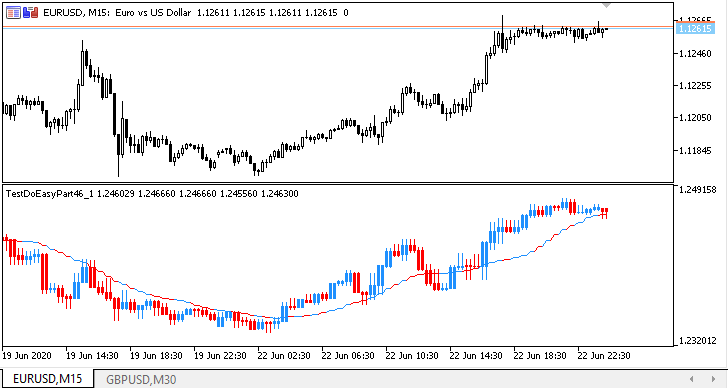For comparison, the GBPUSD chart with the Moving Average indicator having the same parameters was opened.

### Test: multi-period multi-symbol MACD

Now let's create a multi-symbol multi-period MACD. Save the newly created indicator as TestDoEasyPart46_2.mq5.

Set the inputs for MACD and all the necessary buffers for its calculation and display. We will need two drawn buffers: histogram and line to display MACD on the current chart and two calculated ones to store histogram data and MACD signal line obtained from the symbol/period specified in the settings.

I tried to describe in detail all the actions and logic in the comments to the code, so here I will only mention basic changes as compared to the previous indicator:

```//+------------------------------------------------------------------+
//|                                           TestDoEasyPart46_2.mq5 |
//|                        Copyright 2020, MetaQuotes Software Corp. |
//|                             https://mql5.com/en/users/artmedia70 |
//+------------------------------------------------------------------+
#property version   "1.00"
//--- includes
#include <DoEasy\Engine.mqh>
//--- properties
#property indicator_separate_window
#property indicator_buffers 6
#property indicator_plots   2

//--- classes

//--- enums

//--- defines

//--- structures

//--- input variables
sinput   string               InpUsedSymbols    =  "GBPUSD";      // Used symbol (one only)
sinput   ENUM_TIMEFRAMES      InpPeriod         =  PERIOD_M30;    // Used chart period
//---
sinput   uint                 InpPeriodFastEMA  =  12;            // MACD Fast EMA Period
sinput   uint                 InpPeriodSlowEMA  =  26;            // MACD Slow EMA Period
sinput   uint                 InpPeriodSignalMA =  9;             // MACD Signal MA Period
sinput   ENUM_APPLIED_PRICE   InpPriceMACD      =  PRICE_CLOSE;   // MA Applied Price
//---
sinput   bool                 InpUseSounds      =  true;          // Use sounds
//--- indicator buffers
CArrayObj     *list_buffers;                                      // Pointer to the buffer object list
//--- global variables
ENUM_SYMBOLS_MODE    InpModeUsedSymbols=  SYMBOLS_MODE_DEFINES;   // Mode of used symbols list
ENUM_TIMEFRAMES_MODE InpModeUsedTFs    =  TIMEFRAMES_MODE_LIST;   // Mode of used timeframes list
string               InpUsedTFs;                                  // List of used timeframes
CEngine              engine;                                      // CEngine library main object
string               prefix;                                      // Prefix of graphical object names
int                  min_bars;                                    // The minimum number of bars for the indicator calculation
int                  used_symbols_mode;                           // Mode of working with symbols
string               array_used_symbols[];                        // The array for passing used symbols to the library
string               array_used_periods[];                        // The array for passing used timeframes to the library
int                  handle_macd;                                 // МАCD handle
int                  fast_ema_period;                             // Fast EMA calculation period
int                  slow_ema_period;                             // Slow EMA calculation period
int                  signal_period;                               // MACD signal line calculation period
//+------------------------------------------------------------------+
//| Custom indicator initialization function                         |
//+------------------------------------------------------------------+
int OnInit()
{
//--- Write the name of the working timeframe selected in the settings to the InpUsedTFs variable
InpUsedTFs=TimeframeDescription(InpPeriod);
//--- Initialize DoEasy library
OnInitDoEasy();
IndicatorSetInteger(INDICATOR_DIGITS,(int)SymbolInfoInteger(InpUsedSymbols,SYMBOL_DIGITS)+1);
//--- Set indicator global variables
prefix=engine.Name()+"_";
//--- calculate the number of bars of the current period fitting in the maximum used period
//--- Use the obtained value if it exceeds 2, otherwise use 2
int num_bars=NumberBarsInTimeframe(InpPeriod);
min_bars=(num_bars>2 ? num_bars : 2);

//--- Check and remove remaining indicator graphical objects
if(IsPresentObectByPrefix(prefix))
ObjectsDeleteAll(0,prefix);

//--- Create the button panel

//--- Check playing a standard sound using macro substitutions
engine.PlaySoundByDescription(SND_OK);
//--- Wait for 600 milliseconds
engine.Pause(600);
engine.PlaySoundByDescription(SND_NEWS);

//--- indicator buffers mapping
//--- Create all the necessary buffer objects for constructing MACD
engine.BufferCreateHistogram();     // 2 arrays
engine.BufferCreateLine();          // 2 arrays
engine.BufferCreateCalculate();     // 1 array for MACD histogram data from the specified symbol/period
engine.BufferCreateCalculate();     // 1 array for MACD signal line from the specified symbol/period

//--- Check the number of buffers specified in the 'properties' block
if(engine.BuffersPropertyPlotsTotal()!=indicator_plots)
Alert(TextByLanguage("Внимание! Значение \"indicator_plots\" должно быть ","Attention! Value of \"indicator_plots\" should be "),engine.BuffersPropertyPlotsTotal());
if(engine.BuffersPropertyBuffersTotal()!=indicator_buffers)
Alert(TextByLanguage("Внимание! Значение \"indicator_buffers\" должно быть ","Attention! Value of \"indicator_buffers\" should be "),engine.BuffersPropertyBuffersTotal());

//--- Create the color array and set non-default colors to all buffers within the collection
color array_colors[]={clrDodgerBlue,clrRed,clrGray};

//--- Set МАCD calculation periods
fast_ema_period=int(InpPeriodFastEMA<1 ? 1 : InpPeriodFastEMA);
slow_ema_period=int(InpPeriodSlowEMA<1 ? 1 : InpPeriodSlowEMA);
signal_period=int(InpPeriodSignalMA<1  ? 1 : InpPeriodSignalMA);

//--- Get the histogram buffer (the first drawn buffer)
//--- It has the index of 0 considering that the starting point is zero
CBufferHistogram *buff_hist=engine.GetBufferHistogram(0);
if(buff_hist!=NULL)
{
//--- Set the line width for the histogram
buff_hist.SetWidth(3);
//--- Set the graphical series description for the histogram
string label="MACD ("+(string)fast_ema_period+","+(string)slow_ema_period+","+(string)signal_period+")";
buff_hist.SetLabel(label);
//--- and set display in the data window for the buffer
//--- and also a chart period and symbol selected in the settings
buff_hist.SetShowData(true);
buff_hist.SetTimeframe(InpPeriod);
buff_hist.SetSymbol(InpUsedSymbols);
}
//--- Get the signal line buffer (the first drawn buffer)
//--- It has the index of 0 considering that the starting point is zero
CBufferLine *buff_line=engine.GetBufferLine(0);
if(buff_line!=NULL)
{
//--- Set the signal line width
buff_line.SetWidth(1);
//--- Set the graphical series description for the signal line
string label="Signal";
buff_line.SetLabel(label);
//--- and set display in the data window for the buffer
//--- and also a chart period and symbol selected in the settings
buff_line.SetShowData(true);
buff_line.SetTimeframe(InpPeriod);
buff_line.SetSymbol(InpUsedSymbols);
}

//--- Get the first calculated buffer
//--- It has the index of 0 considering that the starting point is zero
CBufferCalculate *buff_calc=engine.GetBufferCalculate(0);
if(buff_calc!=NULL)
{
//--- Set the description of the first calculated buffer as the "MACD histogram temporary array""
buff_calc.SetLabel("MACD_HIST_TMP");
//--- and set a chart period and symbol selected in the settings for it
buff_calc.SetTimeframe(InpPeriod);
buff_calc.SetSymbol(InpUsedSymbols);
}
//--- Get the second calculated buffer
//--- It has the index of 1 considering that the starting point is zero
buff_calc=engine.GetBufferCalculate(1);
if(buff_calc!=NULL)
{
//--- Set the description of the second calculated buffer as the "MACD signal line temporary array""
buff_calc.SetLabel("MACD_SIGN_TMP");
//--- and set a chart period and symbol selected in the settings for it
buff_calc.SetTimeframe(InpPeriod);
buff_calc.SetSymbol(InpUsedSymbols);
}

//--- Display short descriptions of created indicator buffers
engine.BuffersPrintShort();

//--- Create МАCD handle
handle_macd=iMACD(InpUsedSymbols,InpPeriod,fast_ema_period,slow_ema_period,signal_period,InpPriceMACD);
if(handle_macd==INVALID_HANDLE)
return INIT_FAILED;
//---
IndicatorSetString(INDICATOR_SHORTNAME,InpUsedSymbols+" "+TimeframeDescription(InpPeriod)+" MACD("+(string)fast_ema_period+","+(string)slow_ema_period+","+(string)signal_period+")");
//---
return(INIT_SUCCEEDED);
}
//+------------------------------------------------------------------+
//| Custom indicator deinitialization function                       |
//+------------------------------------------------------------------+
void OnDeinit(const int reason)
{
//--- Remove indicator graphical objects by an object name prefix
ObjectsDeleteAll(0,prefix);
Comment("");
}
//+------------------------------------------------------------------+
//| Custom indicator iteration function                              |
//+------------------------------------------------------------------+
int OnCalculate(const int rates_total,
const int prev_calculated,
const datetime &time[],
const double &open[],
const double &high[],
const double &low[],
const double &close[],
const long &tick_volume[],
const long &volume[],
{
//+------------------------------------------------------------------+
//| OnCalculate code block for working with the library:             |
//+------------------------------------------------------------------+
//--- Pass the current symbol data from OnCalculate() to the price structure and set the "as timeseries" flag to the arrays

//--- Check for the minimum number of bars for calculation
if(rates_total<min_bars || Point()==0) return 0;

//--- Handle the Calculate event in the library
//--- If the OnCalculate() method of the library returns zero, not all timeseries are ready - leave till the next tick
if(engine.0)
return 0;

//--- If working in the tester
if(MQLInfoInteger(MQL_TESTER))
{
engine.OnTimer(rates_data);   // Working in the library timer
EventsHandling();             // Working with library events
}
//+------------------------------------------------------------------+
//| OnCalculate code block for working with the indicator:           |
//+------------------------------------------------------------------+
//--- Check and calculate the number of calculated bars
//--- If limit = 0, there are no new bars - calculate the current one
//--- If limit = 1, a new bar has appeared - calculate the first and the current ones
//--- limit > 1 means the first launch or changes in history - the full recalculation of all data
int limit=rates_total-prev_calculated;

//--- Recalculate the entire history
if(limit>1)
{
limit=rates_total-1;
engine.BuffersInitPlots();
engine.BuffersInitCalculates();
}

//--- Prepare data
int total_copy=(limit<2 ? 1 : limit);
//--- Get the first calculated buffer by its number
CBufferCalculate *buff_calc_hist=engine.GetBufferCalculate(0);
//--- Fill in the first calculated buffer with MACD histogram data
int copied=buff_calc_hist.FillAsSeries(handle_macd,0,0,total_copy);
if(copied<total_copy)
return 0;
//--- Get the second calculated buffer by its number
CBufferCalculate *buff_calc_sig=engine.GetBufferCalculate(1);
//--- Fill in the second calculated buffer with MACD signal line data
copied=buff_calc_sig.FillAsSeries(handle_macd,1,0,total_copy);
if(copied<total_copy)
return 0;

//--- Calculate the indicator
CBar *bar=NULL;         // Bar object for defining the candle direction
uchar color_index=0;    // Color index to be set for the buffer depending on the candle direction

//--- Main calculation loop of the indicator
for(int i=limit; i>WRONG_VALUE && !IsStopped(); i--)
{
//--- Clear the current bar of all created buffers
engine.BufferHistogramClear(0,0);
engine.BufferLineClear(0,0);

//--- Get the timeseries bar corresponding to the loop index time on the chart period specified in the settings
bar=engine.SeriesGetBar(InpUsedSymbols,InpPeriod,time[i]);
if(bar==NULL)
continue;
//--- Calculate the color index depending on the candle direction on the timeframe specified in the settings
color_index=(bar.TypeBody()==BAR_BODY_TYPE_BULLISH ? 0 : bar.TypeBody()==BAR_BODY_TYPE_BEARISH ? 1 : 2);
//--- Calculate the MACD histogram buffer
int index=engine.IndexBarPeriodByBarCurrent(i,InpUsedSymbols,InpPeriod);
if(index<0)
continue;
engine.BufferSetDataHistogram(0,i,buff_calc_hist.GetData(index),color_index);
//--- Calculate MACD signal line buffer
engine.BufferSetDataLine(0,i,buff_calc_sig.GetData(index),color_index);
}
//--- return value of prev_calculated for next call
return(rates_total);
}
//+------------------------------------------------------------------+

```

The colors of histogram and signal line columns on each bar correspond to the candle direction of a symbol/period MACD is based on: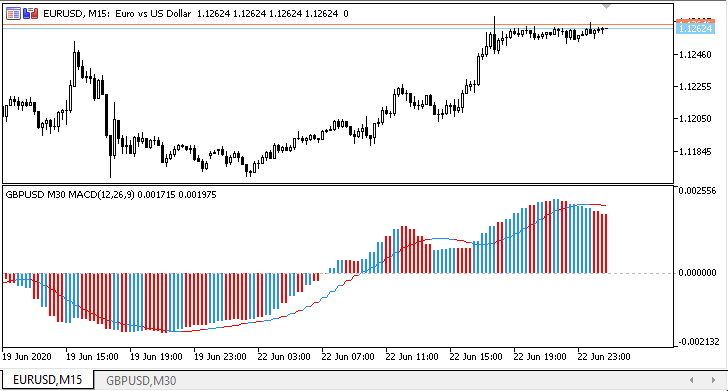The indicator is launched on EURUSD M15 with the default settings of MACD based on GBPUSD M30. The GBPUSD M30 chart with the standard MACD having the same parameters is opened for more clarity.

### What's next?

In the next article, the library will receive the functionality facilitating the creation of multi-symbol multi-period standard indicators.

All files of the current version of the library are attached below together with the test EA files for you to test and download.
Please keep in mind that here I have developed the MQL5 test indicator for MetaTrader 5.
The attached files are intended only for MetaTrader 5. The current library version has not been tested in MetaTrader 4.
After developing and testing the functionality for working with indicator buffers, I will try to implement some MQL5 features in MetaTrader 4.

Back to contents

Previous articles within the series:

Timeseries in DoEasy library (part 35): Bar object and symbol timeseries list
Timeseries in DoEasy library (part 36): Object of timeseries for all used symbol periods
Timeseries in DoEasy library (part 37): Timeseries collection - database of timeseries by symbols and periods
Timeseries in DoEasy library (part 38): Timeseries collection - real-time updates and accessing data from the program
Timeseries in DoEasy library (part 39): Library-based indicators - preparing data and timeseries events
Timeseries in DoEasy library (part 40): Library-based indicators - updating data in real time
Timeseries in DoEasy library (part 41): Sample multi-symbol multi-period indicator
Timeseries in DoEasy library (part 42): Abstract indicator buffer object class
Timeseries in DoEasy library (part 43): Classes of indicator buffer objects
Timeseries in DoEasy library (part 44): Collection class of indicator buffer objects
Timeseries in DoEasy library (part 45): Multi-period indicator buffers

Translated from Russian by MetaQuotes Software Corp.
Original article: https://www.mql5.com/ru/articles/8115

Attached files |
MQL5.zip (3749.86 KB)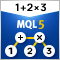Calculating mathematical expressions (Part 2). Pratt and shunting yard parsers

In this article, we consider the principles of mathematical expression parsing and evaluation using parsers based on operator precedence. We will implement Pratt and shunting-yard parser, byte-code generation and calculations by this code, as well as view how to use indicators as functions in expressions and how to set up trading signals in Expert Advisors based on these indicators.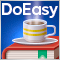Timeseries in DoEasy library (part 45): Multi-period indicator buffers

In this article, I will start the improvement of the indicator buffer objects and collection class for working in multi-period and multi-symbol modes. I am going to consider the operation of buffer objects for receiving and displaying data from any timeframe on the current symbol chart.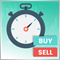Quick Manual Trading Toolkit: Working with open positions and pending orders

In this article, we will expand the capabilities of the toolkit: we will add the ability to close trade positions upon specific conditions and will create tables for controlling market and pending orders, with the ability to edit these orders.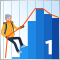Probability theory and mathematical statistics with examples (part I): Fundamentals and elementary theory

Trading is always about making decisions in the face of uncertainty. This means that the results of the decisions are not quite obvious at the time these decisions are made. This entails the importance of theoretical approaches to the construction of mathematical models allowing us to describe such cases in meaningful manner.